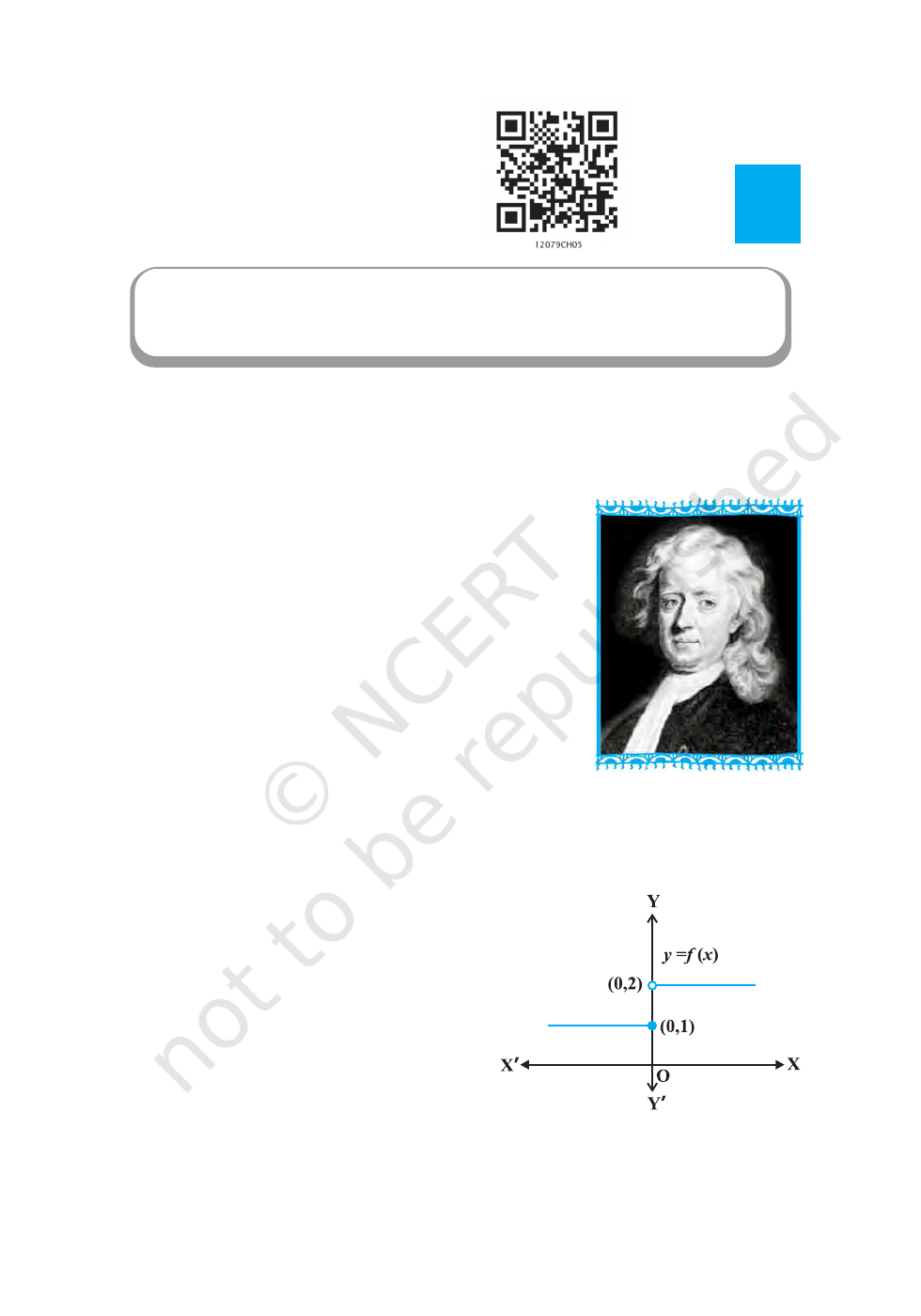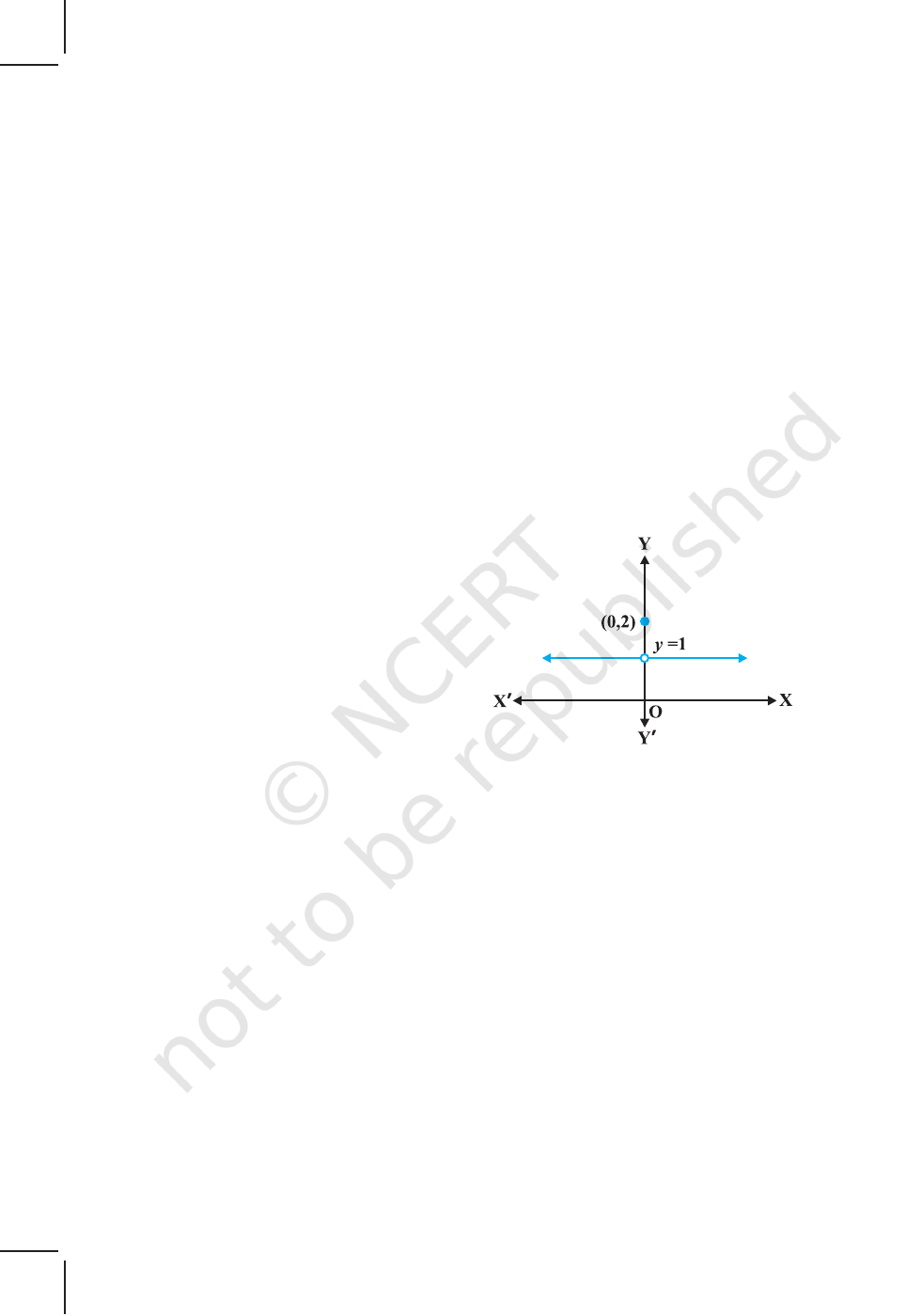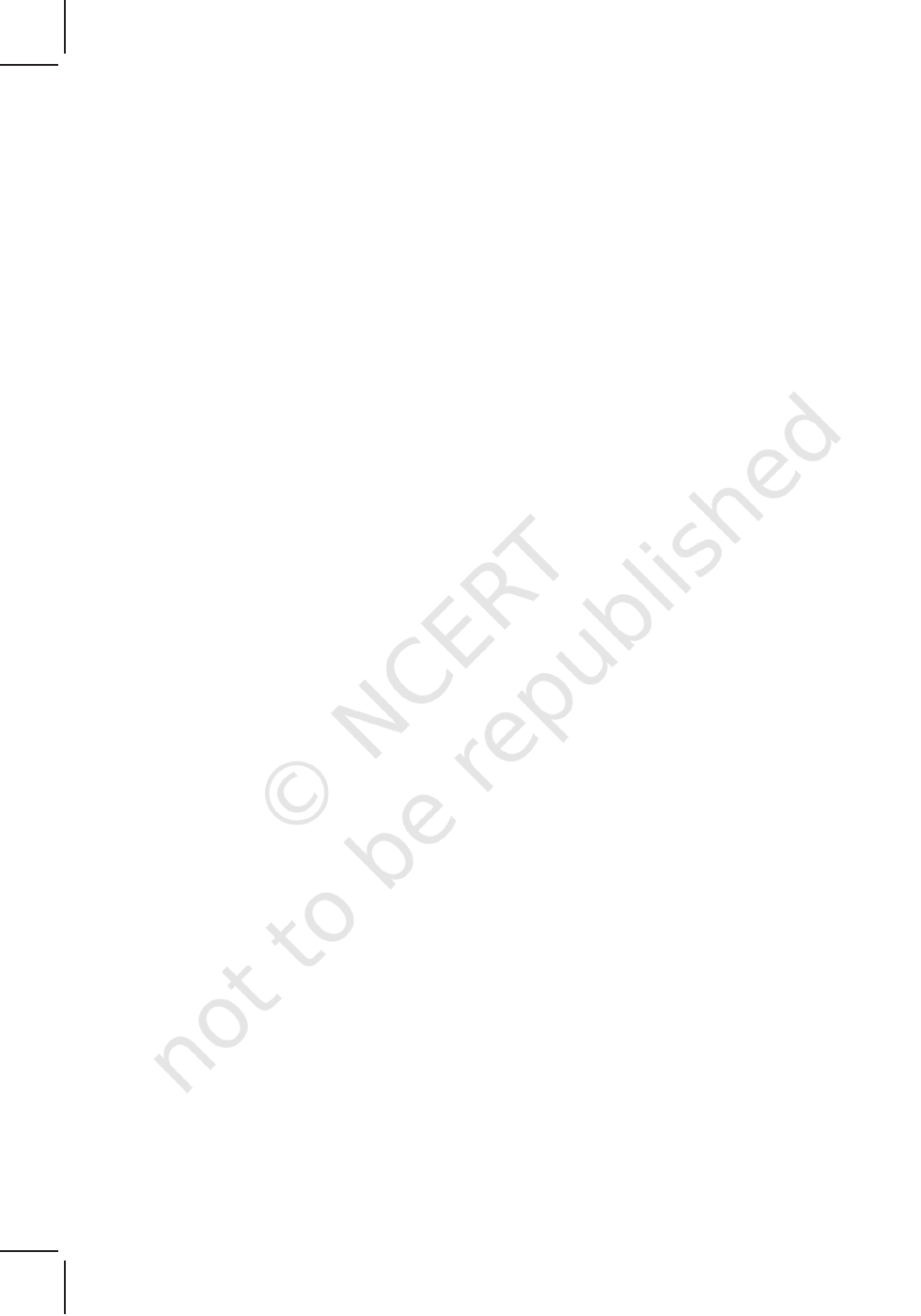v
The whole of science is nothing more than a refinement
of everyday thinking.” — ALBERT EINSTEIN
v
5.1 Introduction
This chapter is essentially a continuation of our study of
differentiation of functions in Class XI. We had learnt to
differentiate certain functions like polynomial functions and
trigonometric functions. In this chapter, we introduce the
very important concepts of continuity, differentiability and
relations between them. We will also learn differentiation
of inverse trigonometric functions. Further, we introduce a
new class of functions called exponential and logarithmic
functions. These functions lead to powerful techniques of
differentiation. We illustrate certain geometrically obvious
conditions through differential calculus. In the process, we
will learn some fundamental theorems in this area.
5.2 Continuity
We start the section with two informal examples to get a feel of continuity. Consider
the function
1, if 0
( )
2, if 0
x
f x
x
=
>
This function is of course defined at every
point of the real line. Graph of this function is
given in the Fig 5.1. One can deduce from the
graph that the value of the function at nearby
points on x-axis remain close to each other
except at x = 0. At the points near and to the
left of 0, i.e., at points like – 0.1, – 0.01, – 0.001,
the value of the function is 1. At the points near
and to the right of 0, i.e., at points like 0.1, 0.01,
Chapter
5
CONTINUITY AND
DIFFERENTIABILITY
Sir Issac Newton
(1642-1727)
Fig 5.1
2019-20MATHEMATICS
148
0.001, the value of the function is 2. Using the language of left and right hand limits, we
may say that the left (respectively right) hand limit of f at 0 is 1 (respectively 2). In
particular the left and right hand limits do not coincide. We also observe that the value
of the function at x = 0 concides with the left hand limit. Note that when we try to draw
the graph, we cannot draw it in one stroke, i.e., without lifting pen from the plane of the
paper, we can not draw the graph of this function. In fact, we need to lift the pen when
we come to 0 from left. This is one instance of function being not continuous at x = 0.
Now, consider the function defined as
f x
x
x
( )
,
,
=
=
1 0
2 0
if
if
This function is also defined at every point. Left and the right hand limits at x = 0
are both equal to 1. But the value of the
function at x = 0 equals 2 which does not
coincide with the common value of the left
and right hand limits. Again, we note that we
cannot draw the graph of the function without
lifting the pen. This is yet another instance of
a function being not continuous at x = 0.
Naively, we may say that a function is
continuous at a fixed point if we can draw the
graph of the function around that point without
lifting the pen from the plane of the paper.
Mathematically, it may be phrased precisely as follows:
Definition 1 Suppose f is a real function on a subset of the real numbers and let c be
a point in the domain of f. Then f is continuous at c if
lim ( ) ( )
f x f c
=
More elaborately, if the left hand limit, right hand limit and the value of the function
at x = c exist and equal to each other, then f is said to be continuous at x = c. Recall that
if the right hand and left hand limits at x = c coincide, then we say that the common
value is the limit of the function at x = c. Hence we may also rephrase the definition of
continuity as follows: a function is continuous at x = c if the function is defined at
x = c and if the value of the function at x = c equals the limit of the function at
x = c. If f is not continuous at c, we say f is discontinuous at c and c is called a point
of discontinuity of f.
Fig 5.2
2019-20CONTINUITY AND DIFFERENTIABILITY 149
Example 1 Check the continuity of the function f given by f(x) = 2x + 3 at
x = 1.
Solution First note that the function is defined at the given point x = 1 and its value is 5.
Then find the limit of the function at x = 1. Clearly
1 1
lim ( ) lim (2 3) 2(1) 3 5
x x
f x x
= + = + =
Thus
1
lim ( ) 5 (1)
x
f x f
= =
Hence, f is continuous at x = 1.
Example 2 Examine whether the function f given by f(x) = x
2
is continuous at x = 0.
Solution First note that the function is defined at the given point x = 0 and its value is 0.
Then find the limit of the function at x = 0. Clearly
2 2
0 0
lim ( ) lim 0 0
x x
f x x
= = =
Thus
0
lim ( ) 0 (0)
x
f x f
= =
Hence, f is continuous at x = 0.
Example 3 Discuss the continuity of the function f given by f(x) = | x | at x = 0.
Solution By definition
f(x) =
, if 0
, if 0
x x
x x
<
Clearly the function is defined at 0 and f(0) = 0. Left hand limit of f at 0 is
0 0
lim ( ) lim (– ) 0
x x
f x x
= =
Similarly, the right hand limit of f at 0 is
0 0
lim ( ) lim 0
x x
f x x
+ +
= =
Thus, the left hand limit, right hand limit and the value of the function coincide at
x = 0. Hence, f is continuous at x = 0.
Example 4 Show that the function f given by
f(x) =
3
3, if 0
1, if 0
x x
x
+
=
is not continuous at x = 0.
2019-20MATHEMATICS
150
Solution
The function is defined at x = 0 and its value at x = 0 is 1. When x 0, the
function is given by a polynomial. Hence,
0
lim ( )
x
f x
=
3 3
0
lim ( 3) 0 3 3
x
x
+ = + =
Since the limit of f at x = 0 does not coincide with f(0), the function is not continuous
at x = 0. It may be noted that x = 0 is the only point of discontinuity for this function.
Example 5 Check the points where the constant function f(x) = k is continuous.
Solution The function is defined at all real numbers and by definition, its value at any
real number equals k. Let c be any real number. Then
lim ( )
x c
f x
=
lim
x c
k k
=
Since f(c) = k =
lim
x c
f(x) for any real number c, the function f is continuous at
every real number.
Example 6 Prove that the identity function on real numbers given by f(x) = x is
continuous at every real number.
Solution The function is clearly defined at every point and f(c) = c for every real
number c. Also,
lim ( )
x c
f x
=
lim
x c
x c
=
Thus,
lim
x c
f(x) = c = f(c) and hence the function is continuous at every real number.
Having defined continuity of a function at a given point, now we make a natural
extension of this definition to discuss continuity of a function.
Definition 2 A real function f is said to be continuous if it is continuous at every point
in the domain of f.
This definition requires a bit of elaboration. Suppose f is a function defined on a
closed interval [a, b], then for f to be continuous, it needs to be continuous at every
point in [a, b] including the end points a and b. Continuity of f at a means
lim ( )
x a
f x
+
= f(a)
and continuity of f at b means
lim ( )
x b
f x
= f(b)
Observe that
lim ( )
x a
f x
and
lim ( )
x b
f x
+
do not make sense. As a consequence
of this definition, if f is defined only at one point, it is continuous there, i.e., if the
domain of f is a singleton, f is a continuous function.
2019-20